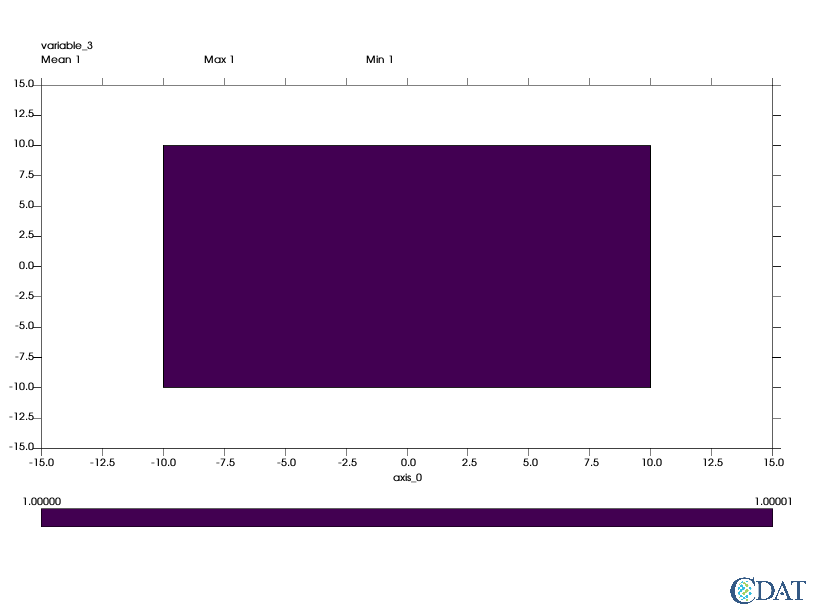{{ message }}

# meshfill wrap seems broken#323

Closed
opened this issue Mar 2, 2018 · 0 comments
Closed

# meshfill wrap seems broken#323

opened this issue Mar 2, 2018 · 0 comments
Labels
Milestone

###doutriaux1 commented Mar 2, 2018

 ```import vcs import numpy data = numpy.array() lats = numpy.array([0.]) lons = numpy.array([0.]) blats = [-10,-10,10,10] blons = [-10,10,10,-10] mesh = numpy.zeros((1,2,4)) mesh[:,0] = blats[:] mesh[:,1] = blons[:] x = vcs.init() gm = vcs.createmeshfill() gm.datawc_x1 = -15 gm.datawc_x2 = 15 gm.datawc_y1 = -15 gm.datawc_y2 = 15 gm.mesh = True gm.wrap = [0.,20.] x.plot(data,mesh,gm)``` should plot 3 cells but generatesThe text was updated successfully, but these errors were encountered:
added a commit that referenced this issue Mar 2, 2018
``` seems to fix #323 ```
``` ad27cd3 ```